Ex 4.1

Chapter 4 Class 11 Mathematical Induction
Serial order wise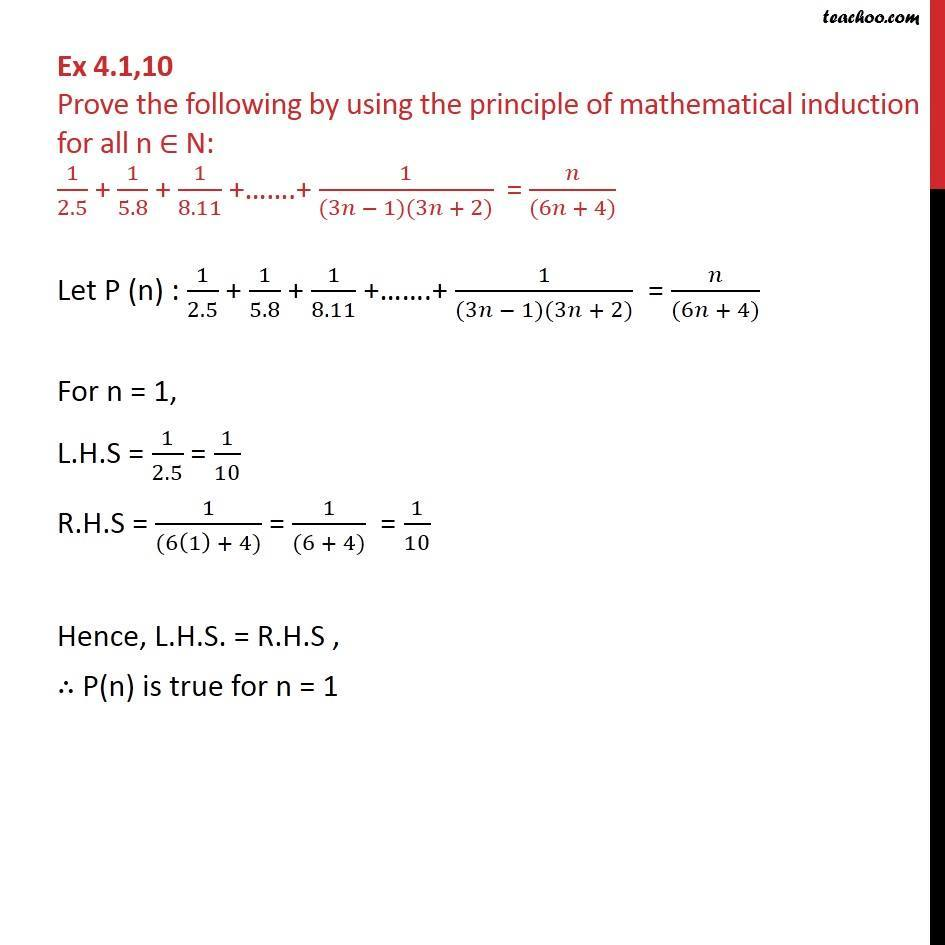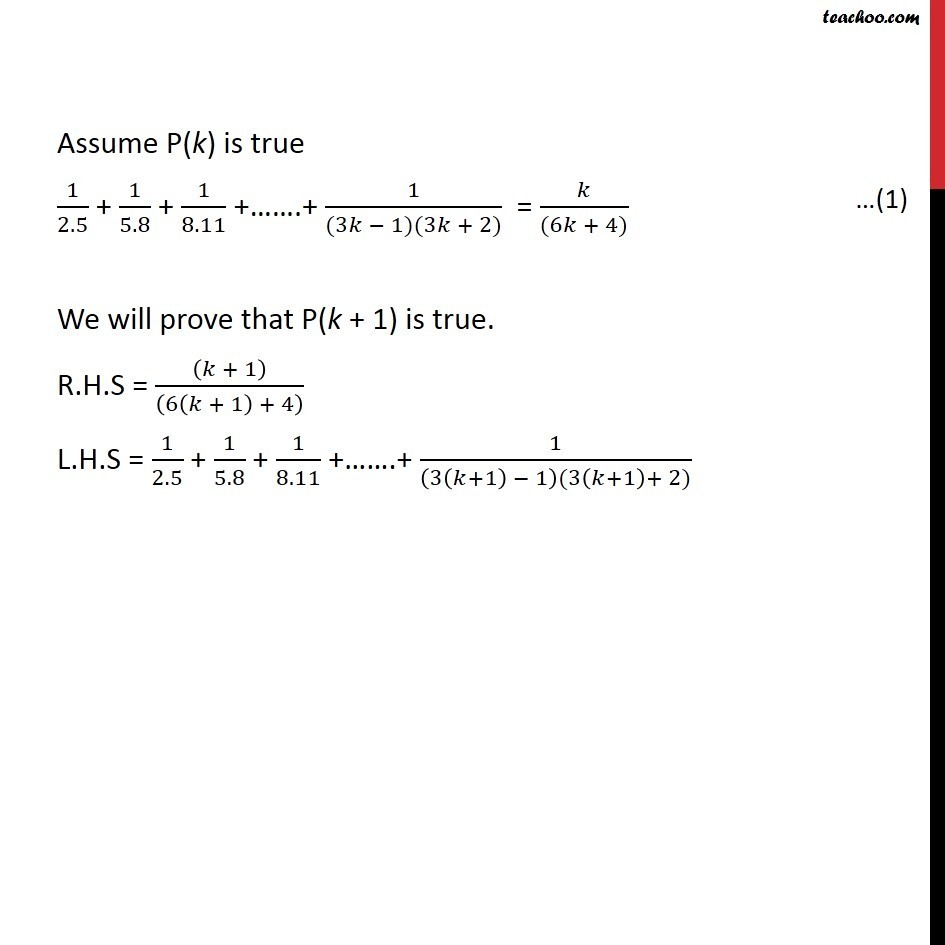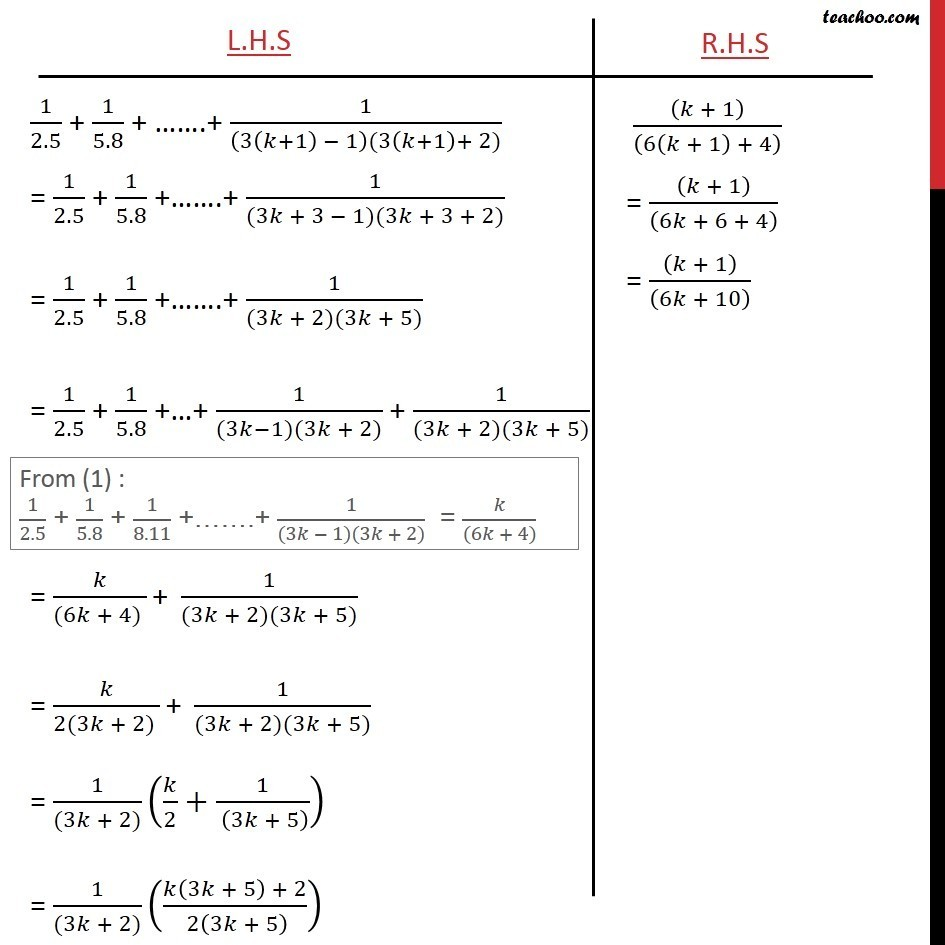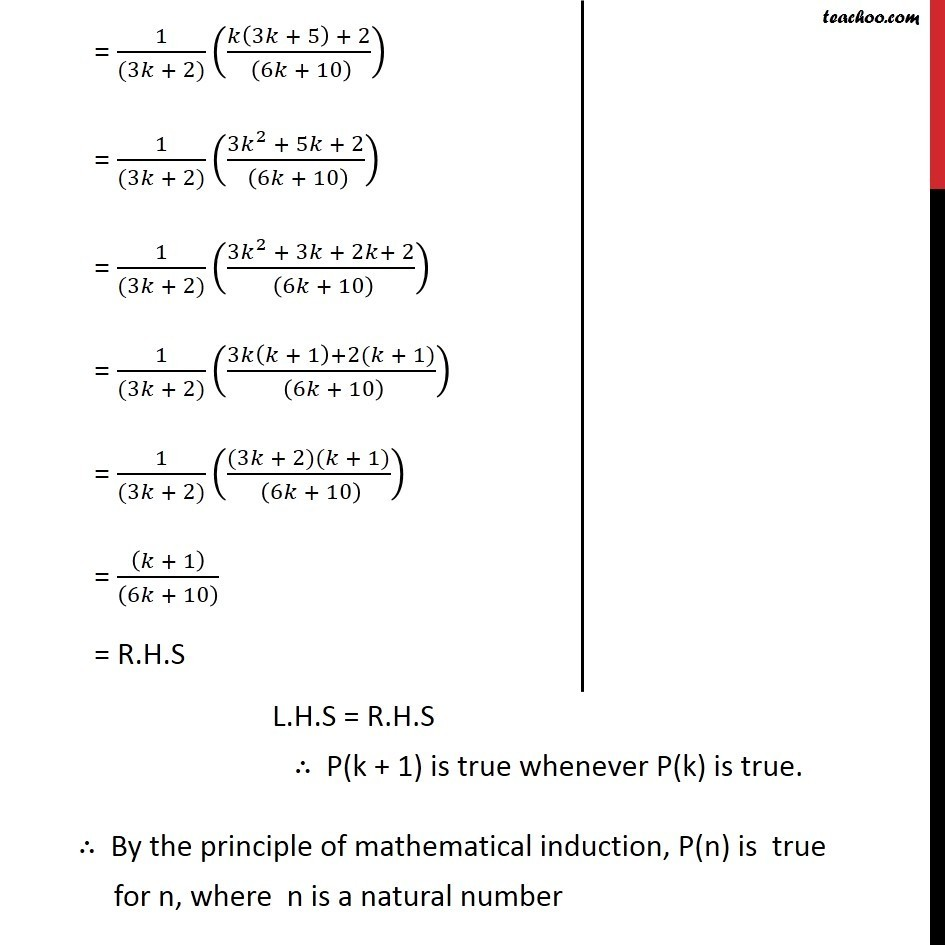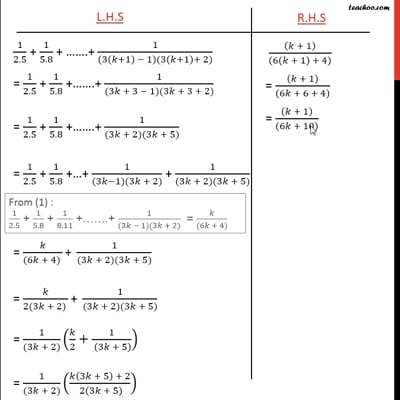This video is only available for Teachoo black users

Solve all your doubts with Teachoo Black (new monthly pack available now!)

### Transcript

Ex 4.1,10 Prove the following by using the principle of mathematical induction for all n N: 1/2.5 + 1/5.8 + 1/8.11 + .+ 1/((3 1)(3 + 2)) = /((6 + 4)) Let P (n) : 1/2.5 + 1/5.8 + 1/8.11 + .+ 1/((3 1)(3 + 2)) = /((6 + 4)) For n = 1, L.H.S = 1/2.5 = 1/10 R.H.S = 1/((6(1) + 4)) = 1/((6 + 4)) = 1/10 Hence, L.H.S. = R.H.S , P(n) is true for n = 1 Assume P(k) is true 1/2.5 + 1/5.8 + 1/8.11 + .+ 1/((3 1)(3 + 2)) = /((6 + 4)) We will prove that P(k + 1) is true. R.H.S = (( + 1))/((6( + 1) + 4) ) L.H.S = 1/2.5 + 1/5.8 + 1/8.11 + .+ 1/((3( +1) 1)(3( +1)+ 2))